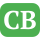CppBuzz.com

# Write C++ programs to perform the following task

```A.Input and output your name,address and age to an appropriate structure

B.Write a program to read a "float" representing a number of degrees Celsius and print as a "float" the equivalent temperature in degrees Fahrenheit Print your results in a form such as

100 0 degrees Celsius converts to 212 0 degrees Fahrenheit

C.Given as Input a floating (real) number to centimeters print the equivalent number of feet (interger) and inches (floating, 1 decimal)with the inches given an accuracy of one decimal place

Assume 2.54 centimeters per inch and 12 inch per foot
if the input value is 333.3 centimeters the output value should be:
333.3 centimeters is 10 feet 11.2 inches

D.Given as input an interger number of seconds print as output the equivalent time in hours,minutes,seconds Reccomend output format is something like 7322 seconds equivalent to 2 hours 2minutes 2 seconds.

i hope you can help me i pass it tommorow :)```

Posted by uehara123 2019-01-24 03:50:51

```Hi,Admin posted 2019-01-24 14:44:14